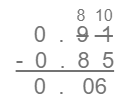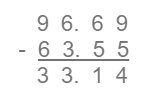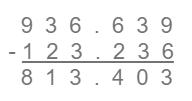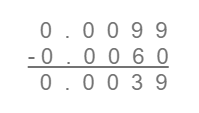### Decimal Numbers - Worksheets

CBSE Worksheet-01

Class - IV Mathematics – Decimal Numbers

Time Allowed: 30 minutes

1. Fill in the blanks with suitable words:
1. In 0.87 , 8 stands for  ___________
2. A __________ is a fraction whose denominator is 10 or power of ten.
3. Place value of 7 in 12.075 is ___________
4. The decimal number 2.5 is read as __________
5. The decimal numeral for Nine thousandths is ________
2. State whether each of the following statement is True or False:
1. Eight and sixty-seven hundredth can be written as 8.067
2. $6\frac{3}{10}$ can be written as 6.3
3. 12.36 can be written in fraction as $12\frac{3}{6}$.
4. Hundredth place of 5.28 is 0.08.
5. Fraction form of 0.01 is $\frac{1}{10}$.
3. The place value of 3 in 4.375 is
1. $\frac{3}{1000}$
2. 3
3. $\frac{3}{10}$
4. 30
4. Fill the box 0.538 = $\frac{5}{10}+\frac{3}{100}+\frac{8}{?}$
1. 10000
2. 100
3. 10
4. 1000
5. Write the number in expanded form: 765.149
1. $700+60+5+\frac{1}{100}+\frac{4}{1000}+\frac{9}{1000}$
2. $700+65+\frac{1}{10}+\frac{4}{100}+\frac{9}{1000}$
3. $760+5+\frac{1}{10}+\frac{4}{100}+\frac{9}{1000}$
4. $700+60+5+\frac{1}{10}+\frac{4}{100}+\frac{9}{1000}$
6. 5.7 is equal to
1. $\frac{5}{10}$
2. $\frac{57}{10}$
3. $\frac{5}{7}$
4. $\frac{57}{100}$
7. $\frac{4}{10}=\mathrm{_}\mathrm{_}\mathrm{_}\mathrm{_}$
1. None of these
2. 4
3. 0.4
4. 40
8. Match the following:

 (a) nine tenths (i) 61000$\frac{6}{1000}$ (b) Twenty-five hundredth (ii) 1910$1\frac{9}{10}$ (c) Six thousandth (iii) 25100$\frac{25}{100}$ (d) one and nine tenth (iv) 910$\frac{9}{10}$
9. Subtract:

1. 5.158 from 7.9
2. 0.00084 kg from 0.05 kg
10. In a dance competition, Ritu got 8.5 points from 1st judge, 7.75 points from 2nd judge, and 9.03 points from 3rd judge. How many points did Ritu get? If Sakshi gets 1.75 points more than Ritu then how many points did Sakshi get?

11. Convert the following decimal to fractions.

1. 0.999
2. 21.23
12. Write as common fractions:
0.45, 13.09, 6.007, 5.1, 0.352, 44.4

13. Arrange the following decimal numbers in descending order.

1. ​​​​​2.23, 2.43, 2.33, 2.53
2. 4.45, 5.44, 8.40, 7.40
3. 1.29, 2.29, 1.39, 2.39
14. Subtract:

1. 0.91 from 0.85
2. 63.55 from 96.69
3. 123.236 from 936.639
4. 0.006 from 0.0099
15. Write the following as decimals:

1. 6000 + 20 + $\frac{1}{10}$ + $\frac{7}{1000}$
2. 8000 + 2 + $\frac{1}{100}$
3. 200000 + 600 + $\frac{5}{100}$ + $\frac{5}{1000}$
4. 10000 + 60 + 2 + $\frac{5}{10}$ + $\frac{4}{100}$ + $\frac{3}{1000}$
16. Convert each of the following as decimal.

1. $\frac{6}{5}$
2. $\frac{9}{25}$
3. $\frac{57}{250}$
4. $\frac{61}{25}$

CBSE Worksheet-01
Class - IV Mathematics – Decimal Numbers

Solution

1. Fill in the blanks with suitable words:

1. 8 tenths

2. Decimal.

3. 7/100

10+2+(0/10)+(7/100)+(5/1000) is the expanded form

4. Two point five

5. 0.009

2. State whether each of the following statement is True or False:

1. False, Eight and sixty-seven hundredth can be written as 8.67

2. True, $6\frac{3}{10}$ = 6 + 0.3 = 6.3

3. False, 12.36 can be written in fraction as $12\frac{36}{100}$.

4. True, Hundredth place of 5.28 is 0.08.

5. True, 0.01 = $\frac{1}{10}$.

3. (c) $\frac{3}{10}$
Explanation: Place value of 3 in 4.375 is $\frac{3}{10}$.
3 is in tenths place so its value is $\frac{3}{10}$.
4. (d) 1000
Explanation:

0.538 = $\frac{5}{10}+\frac{3}{100}+\frac{8}{1000}$

8 is in thousandths place so $\frac{1}{1000}$.

5. (d) $700+60+5+\frac{1}{10}+\frac{4}{100}+\frac{9}{1000}$
Explanation:
Place value of 7 = 7 × 100 = 700
Place value of 6 = 6 × 10 = 60
Place value of 1 = 1 × 1 = 1
Place value of 4 = 4 × $\frac{1}{100}$  = $\frac{4}{100}$
Place value of 9 = 9 × $\frac{1}{1000}$  = $\frac{9}{1000}$
So , 765.149 = $700+60+5+\frac{1}{10}+\frac{4}{100}+\frac{9}{1000}$
6. (b) $\frac{57}{10}$
Explanation: $\frac{57}{10}$
57 $÷$ 10 = 5.7
57 $÷$ 100 = 0.57
$÷$ 7 = 0.71
So, the correct answer is $\frac{57}{10}$
7. (c) 0.4
Explanation: $\frac{4}{10}=0.4$

In 0.4, 4 is in tenths place so $\frac{4}{10}=0.4$.

8. (a) – (iv), (b) – (iii), (c) – (i), (d) – (ii)

1. 5.158 from 7.9
7.9 - 5.158 = 2.742
2. 0.00084 kg from 0.05 kg
0.05 - 0.00084 = 0.04916
9. Total point Ritu got in competition = 8.50 + 7.75 + 9.03 = 25.28 points
then, point Sakshi got = 25.28 + 1.75 = 27.03 points

1. 0.999
$=\frac{9}{10}+\frac{9}{100}+\frac{9}{1000}$
$=\frac{900}{1000}+\frac{90}{1000}+\frac{9}{1000}$
$\frac{999}{1000}$
2. 21.23
= 21 + 0.23
= 21 + $\frac{2}{10}+\frac{3}{100}$
= 21 + $\frac{20}{100}+\frac{3}{100}$
$21+\frac{23}{100}$
$21\frac{23}{100}$
1. 0.45
We can write it as $\frac{0.45}{1}$
then, multiply numerator and denominator by 100
now, $\frac{0.45}{1}$ $×$ $\frac{100}{100}$ = $\frac{45}{100}$
so, the common fraction is $\frac{45}{100}$
2. 13.09
We can write it as $\frac{13.09}{1}$
then, multiply numerator and denominator by 100
now, $\frac{13.09}{1}$ $×$ $\frac{100}{100}$ = $\frac{1309}{100}$
so, the common fraction is $\frac{1309}{100}$
3. 6.007
We can write it as $\frac{6.007}{1}$
then, multiply numerator and denominator by 1000
now, $\frac{6.007}{1}$ $×$ $\frac{1000}{1000}$ = $\frac{6007}{1000}$
so, the common fraction is $\frac{6007}{1000}$
4. 5.1
We can write it as $\frac{5.1}{1}$
then, multiply numerator and denominator by 10
now, $\frac{5.1}{1}$ $×$ $\frac{10}{10}$ = $\frac{51}{10}$
so, the common fraction is $\frac{51}{10}$
5. 0.352
We can write it as $\frac{0.352}{1}$
then, multiply numerator and denominator by 1000
now, $\frac{0.352}{1}$$×$$\frac{1000}{1000}$ = $\frac{352}{1000}$
so, the common fraction is $\frac{352}{1000}$
6. 44.4
We can write it as $\frac{44.4}{1}$
then, multiply numerator and denominator by 10
now, $\frac{44.4}{1}$ $×$ $\frac{10}{10}$ = $\frac{444}{10}$
so, the common fraction is $\frac{444}{10}$
1. ​​​​​2.23, 2.43, 2.33, 2.53
Here, the one's place is 2 for all decimal numbers
0.53 > 0.43 > 0.33 > 0.23
2.53 > 2.43 > 2.33 > 2.23
2. 4.45, 5.44, 8.40, 7.40
Here, one's place is different for all decimal numbers
8 > 7 > 5 > 4
8.40 > 7.40 > 5.44 > 4.45
3. 1.29, 2.29, 1.39, 2.39
Here,
As hundredths place is same for all numbers:
2>1 and  39 > 29
2.39 > 2.29 > 1.39 > 1.29
1. 0.85 from 0.91 = 0.062. 63.55 from 96.69 = 33.143. 123.236 from 936.639 = 813.4034. 0.006 from 0.0099 = 0.00391. 6000 + 20 + $\frac{1}{10}$ + $\frac{7}{1000}$
6000 + 20 + 0.1 + 0.007 = 6020.107
2. 8000 + 2 + $\frac{1}{100}$
8000 + 2 + 0.01 = 8002.01
3. 200000 + 600 + $\frac{5}{100}$ + $\frac{5}{1000}$
200000 + 600 + 0.05 + 0.005 = 200600.055
4. 10000 + 60 + 2 + $\frac{5}{10}$ + $\frac{4}{100}$ + $\frac{3}{1000}$
10000 + 60 + 2 + 0.5 + 0.04 + 0.003 = 10062.543
1. $\frac{6}{5}$
$\frac{6×2}{5×2}=\frac{12}{10}$ = 1.2
2. $\frac{9}{25}$
$\frac{9×4}{25×4}=\frac{36}{100}=0.36$
3. $\frac{57}{250}$
$\frac{57×4}{250×4}=\frac{228}{1000}=0.228$
4. $\frac{61}{25}$